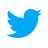# Learn to flatten array in JavaScript with one liners

#javascript#programming#arrays
January 31, 2022

In JavaScript, an array is a collection of ordered elements and there's no restriction for the type of elements.

An array is said to be flat or flattened if there are no nested arrays inside the array.

Let's see what an array and a flattened array look like with an example.

``````// An array with nested arrays
const letters = ['a', 'b', ['c', 'd'], ['e', 'f']];

// Flattened array
["a", "b", "c", "d", "e", "f"]
``````

In this article, we are going to see different ways to flatten arrays in JavaScript.

## Flatten arrays in JS

We'll see different ways to flatten this `letters` array in JS.

``const letters = ['a', 'b', ['c', 'd'], ['e', 'f']];``

### 1. Using concat() array method

`concat()` array method is used to merge two or more arrays. It takes a value or an array as an argument and concatenates it to the original array and returns the result as a new array without mutating the original one.

We'll use an empty array and concatenate the `letters` array elements with the help of a spread operator to spread the nested array elements.

``````const letters = ['a', 'b', ['c', 'd'], ['e', 'f']];

const flatArray = [].concat(...letters);

// flatArray: ['a', 'b', 'c', 'd', 'e', 'f'];``````

### 2. Using concat() array method with apply()

We can use `apply()` method on `concat` function as apply method helps to pass arguments in the form of an array which we have already seen in call, apply, bind Javascript article.

So the idea is to pass every individual element of the array to the `concate` method instead of the whole array.

In the previous method, we have used the `...` spread operator for the same reason.

``````const letters = ['a', 'b', ['c', 'd'], ['e', 'f']];

const flatArray = [].concat.apply([], letters);

// flatArray: ['a', 'b', 'c', 'd', 'e', 'f'];``````

The first array need not be empty and could be anything in `[].concat` because the array that we pass as the first argument to `apply()` will be considered for creating the new array.

### 3. Using reduce() array method

We can use `reduce()` method to flatten an array as flattening is a way of reducing the original array to a different form.

The idea is to spread every element and nested element in a new array and return the result, and the result is nothing but the flattened array.

``````const letters = ['a', 'b', ['c', 'd'], ['e', 'f']];

const flatArray = letters.reduce((acc, curr) => [...acc, ...curr], []);

// flatArray: ['a', 'b', 'c', 'd', 'e', 'f'];``````

We can omit the array given as a second argument to the `reduce` method, it is just to initialize the accumulator with an empty array.

### 4. Using the flat() array method

There's a new array method called `flat()` added in the ECMAScript version 2019 or ES10. What it does is simply flattens the array when it is called.

Using `flat()` method we can flatten arrays of any depth by passing the max. depth to be flattened to as an argument to the flat method. The default depth is 1.

``````const letters = ['a', 'b', ['c', 'd'], ['e', 'f']];

const flatArray = letters.flat(); // default depth 1

// flatArray: ['a', 'b', 'c', 'd', 'e', 'f'];``````

To flatten an array of depth greater than 1.

``````const letters = ['a', 'b', ['c', 'd', ['e', 'f']]];

const flatArray = letters.flat(2); // Flatten until depth 2

// flatArray: ['a', 'b', 'c', 'd', 'e', 'f'];``````

To flatten any amount of depth, we can pass `Infinity` as the argument to the `flat()` method.

### 5. By converting to string and splitting

Arrays of any depth when converted to a string gets flattened and will be joined by `,`(comma).

So using this technique after converting an array to a string and then splitting it by `,` we can flatten the array.

``````const letters = ['a', 'b', ['c', 'd'], ['e', 'f']];

const flatArray = letters.toString().split(',');

// flatArray: ['a', 'b', 'c', 'd', 'e', 'f'];``````

The main problem with this method is that it works well when all the elements in the array are strings or of any one specific type, say numbers. Because after generating the array we can convert it to a specific type.

But when the array consists of multiple type elements then it gets difficult to use this method.

Let's see how an array of numbers can be flattened using this technique.

``````const numbers = [1, 2, [3, 4], [5, 6]];

const flatArray = numbers.toString().split(',').map(Number);
// or const flatArray = numbers.join().split(',').map(Number);
// or const flatArray = `\${numbers}`.split(',').map(Number);

// flatArray: [1, 2, 3, 4, 5, 6]``````

And that is all about different ways to flatten Javascript arrays. Most of the browsers have support for `flat()` method so it would be handy to use this method in most of the scenarios.

If you have any other way to flatten the arrays in a better manner then do share it with us and we'll be happy to update this article with that.

If you like receiving regular tips, tricks related to web development and technology then do follow on@dev_apt